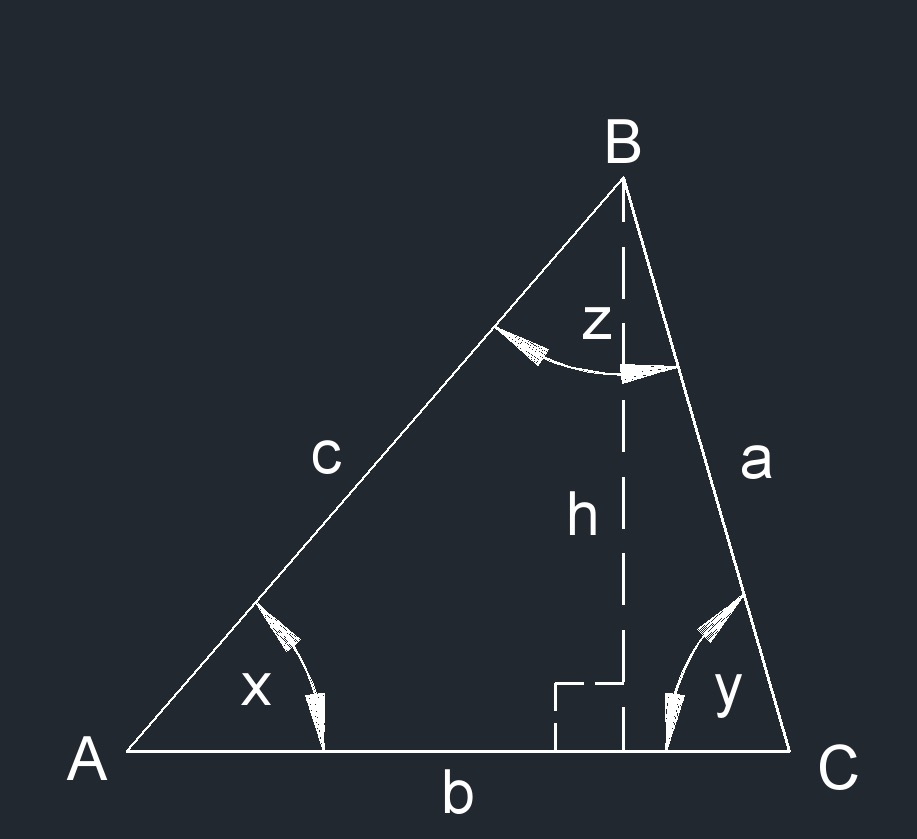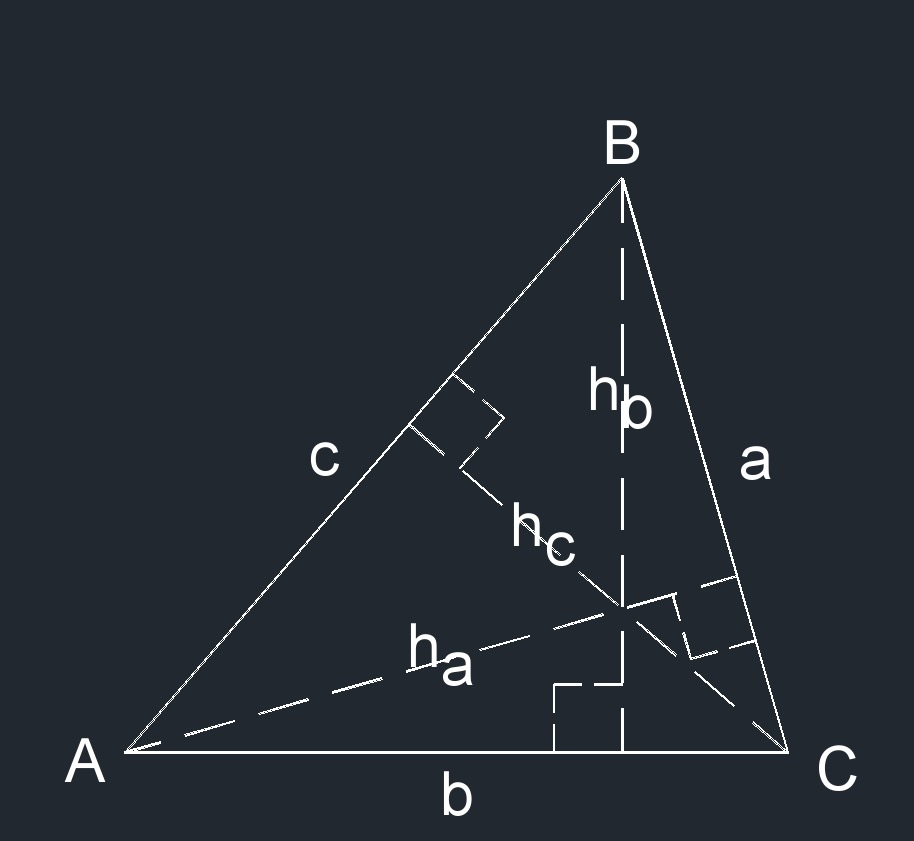# Scalene Triangle

Written by Jerry Ratzlaff on . Posted in Plane Geometry

•Scalene triangle (a two-dimensional figure) is where all three sides are different lengths and all three angles are different angles.
• Angle bisector of a scalene triangle is a line that splits an angle into two equal angles.
• Median of a scalene triangle is a line segment from a vertex (coiner point) to the midpoint of the opposite side.
• Circumcircle is a circle that passes through all the vertices of a two-dimensional figure.
• Inscribed circle is the Iargest circle possible that can fit on the inside of a two-dimensional figure.
• Semiperimeter is one half of the perimeter.
• x + y + z = 180°
• Height:  $$h_a$$,  $$h_b$$,  $$h_c$$
• Median:  $$m_a$$,  $$m_b$$,  $$m_c$$  -  A line segment from a vertex (corner point) to the midpoint of the opposite side
• Angle bisectors:  $$t_a$$,  $$t_b$$,  $$t_c$$  -  A line that splits an angle into two equal angles
• 3 edges
• 3 vertexs## Formulas that use Angle bisector of a Scalene Triangle

 $$\large{ t_a = 2\;b \;c \; cos \; \frac { \frac {A}{2} } { b \;+\; c } }$$ $$\large{ t_a = \sqrt { b\;c \; \frac { 1 \;- \; a^2 }{ \left( b \;+\; c \right)^2 } } }$$

### Where:

$$\large{ t_a }$$ = angle bisector

$$\large{ A }$$ = angle

$$\large{ a, b, c }$$ = edge

## Formulas that use Area of a Scalene Triangle

 $$\large{ A_{area} = \frac {h\;b} {2} }$$ $$\large{ A_{area} = a\;b\; \frac {\sin y} {2} }$$

### Where:

$$\large{ A_{area} }$$ = area

$$\large{ a, b, c }$$ = edge

## Formulas that use Circumcircle of a Scalene Triangle

 $$\large{ R = \sqrt { \frac { a^2 \; b^2 \; c^2 } { \left( a \;+\; b \;+\; c \right) \; \left( - a + b + c \right) \; \left( a \;-\; b \;+\; c \right) \; \left( a \;+\; b \;-\; c \right) } } }$$ $$\large{ R = \frac { a \; b \; c } { 4 \; \sqrt { s\; \left( s \;-\; a \right) \; \left( s \;-\; b \right) \; \left( s \;-\; c \right) } } }$$

### Where:

$$\large{ R }$$ = outcircle

$$\large{ a, b, c }$$ = edge

$$\large{ s }$$ = semiperimeter

## Formulas that use Height of a Scalene Triangle

 $$\large{ h_a = c \; sin\; B }$$ $$\large{ h_a = b \; sin\; C }$$ $$\large{ h_a = 2\; \frac {A_{area}}{a} }$$

### Where:

$$\large{ h_a }$$ = height

$$\large{ a, b, c }$$ = edge

$$\large{ B, C }$$ = angle

$$\large{ A_{area} }$$ = area

## Formulas that use Inscribed Circle of a Scalene Triangle

 $$\large{ r = \sqrt { \frac { \left( s \;-\; a \right) \; \left( s \;-\; b \right) \; \left( s \;-\; c \right) } { s } } }$$

### Where:

$$\large{ r }$$ = incircle

$$\large{ a, b, c }$$ = edge

## Formulas that use Median of a Scalene Triangle

 $$\large{ m_a = \sqrt { \frac { 2\;b^2 \;+\; 2\;c^2 \;-\; a^2 } {2} } }$$

### Where:

$$\large{ m_a }$$ = median

$$\large{ a, b, c }$$ = edge

## Formulas that use Perimeter of a Scalene Triangle

 $$\large{ P = a + b + c }$$

### Where:

$$\large{ P }$$ = perimeter

$$\large{ a, b, c }$$ = edge

## Formulas that use Semiperimeter of a Scalene Triangle

 $$\large{ s = \frac{ a \;+\; b \;+\; c }{ 2 } }$$

### Where:

$$\large{ s }$$ = semiperimeter

$$\large{ a, b, c }$$ = edge

## Formulas that use Side of a Scalene Triangle

 $$\large{ a = P - b - c }$$ $$\large{ a = 2\; \frac {A_{area}} {b\;\sin y} }$$ $$\large{ b = P - a - c }$$ $$\large{ b = 2\; \frac {A_{area}}{h} }$$ $$\large{ c = P - a - b }$$

### Where:

$$\large{ a, b, c }$$ = edge

$$\large{ P }$$ = perimeter

$$\large{ A_{area} }$$ = area